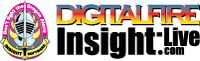•The secret to cool bodies and glazes is a lot of testing.
•The secret to know what to test is material and chemistry knowledge.
•The secret to learning from testing is documentation.
•The place to test, do the chemistry and document is an account at https://insight-live.com
•The place to get the knowledge is https://digitalfire.com

Sign-up at https://insight-live.com today.

# SX Blue Ball Clay

 Oxide Analysis Formula CaO 0.24% 0.012 MgO 0.24% 0.017 K2O 0.60% 0.018 Na2O 0.56% 0.026 TiO2 0.71% 0.026 Al2O3 35.52% 1.000 SiO2 45.65% 2.181 Fe2O3 1.25% 0.022 LOI 15.22 Oxide Weight 243.43 Formula Weight 287.13

## XML for Import into INSIGHT

<?xml version="1.0" encoding="UTF-8"?> <material name="SX Blue Ball Clay" descrip="" searchkey="" loi="15.22" casnumber="70694-09-6"> <oxides> <oxide symbol="CaO" name="Calcium Oxide, Calcia" status="" percent="0.240" tolerance=""/> <oxide symbol="MgO" name="Magnesium Oxide, Magnesia" status="" percent="0.240" tolerance=""/> <oxide symbol="K2O" name="Potassium Oxide" status="" percent="0.600" tolerance=""/> <oxide symbol="Na2O" name="Sodium Oxide, Soda" status="" percent="0.560" tolerance=""/> <oxide symbol="TiO2" name="Titanium Dioxide, Titania" status="" percent="0.710" tolerance=""/> <oxide symbol="Al2O3" name="Aluminum Oxide, Alumina" status="" percent="35.520" tolerance=""/> <oxide symbol="SiO2" name="Silicon Dioxide, Silica" status="" percent="45.650" tolerance=""/> <oxide symbol="Fe2O3" name="Iron Oxide, Ferric Oxide" status="" percent="1.250" tolerance=""/> </oxides> <volatiles> <volatile symbol="LOI" name="Loss on Ignition" percent="15.220" tolerance=""/> </volatiles> </material>